Graph of a function of multiple variables

Definition

Suppose$f$ is a real-valued function of$n$ variables$x_1,x_2,\dots,x_n$. The graph of$f$ is a subset of$\R^{n+1}$, with coordinate axes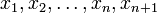$x_1,x_2,\dots,x_n,x_{n+1}$, given as follows: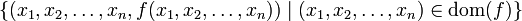$\{ (x_1,x_2,\dots,x_n,f(x_1,x_2,\dots,x_n)) \mid (x_1,x_2,\dots,x_n) \in \operatorname{dom}(f)\}$

where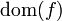$\operatorname{dom}(f)$ denotes the domain of$f$.

Alternatively, it is given by the equation: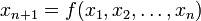$x_{n+1} = f(x_1,x_2,\dots,x_n)$

For nice enough functions, this graph looks like a hypersurface of codimension one (and dimension$n$) inside$\R^{n+1}$.

Aspects

Domain and range

Aspect of the function How it can be deduced from the graph
domain project the entire graph on the$x_1x_2 \dots x_n$-hyperplane
range project the entire graph on the$x_{n+1}$-axis

Vertical line test

The vertical line test for a function of one variable says that every vertical line intersects the graph in exactly one point if the$x$-coordinate is in the domain and in no point if the$x$-coordinate is not in the domain. There is an analogous test for a function of multiple variables. This says that for any line parallel to the$x_{n+1}$-axis, the intersection with the graph has size one (if the intersection with the$x_1x_2\dots x_n$-hyperplane is in the domain) or zero (if it isn't).

Restriction to one variable

Graph of the restriction

We can restrict the function to a function of fewer variables by fixing the value of some of the variables. Suppose$A$ is a subset of \{ 1,2,\dots,n\}[/itex]. Suppose we fix the values of the coordinates in$A$, so we get$x_j = a_j$ for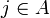$j \in A$.

This is now a function of the remaining variables, which is a total of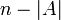$n - |A|$ variables.

The graph of this function is obtained by intersecting the original graph with the affine subspace given by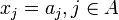$x_j = a_j, j \in A$. Note that this subspace has dimension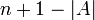$n + 1 - |A|$, and the intersection of the graph with this is expected to have dimension$n - |A|$.

Here's an extreme case:$A$ has size$n - 1$, and the only variable omitted is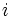$i$. Then, the function we obtain is a function of one variable: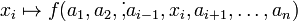$x_i \mapsto f(a_1,a_2,\dot,a_{i-1},x_i,a_{i+1},\dots,a_n)$

The graph of this is obtained by intersecting the original graph with the plane given by equations$x_j = a_j$ for all$j \ne i$. There are$n - 1$ such equations. The plane itself is parallel to the$x_ix_{n+1}$-plane.

Continuity in each variable and separate continuity in graphical terms

We have the following:

Assertion about continuity How we can verify it from the graph$f$ is continuous in$x_i$ at the point$(a_1,a_2,\dots,a_n)$ Consider the graph restricted to the plane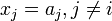$x_j = a_j, j \ne i$. This graph is continuous at$x_i = a_i$.$f$ is separately continuous in all variables at the point$(a_1,a_2,\dots,a_n)$. The above holds for all$i \in \{ 1,2,3,\dots,n\}$.$f$ is continuous in$x_i$ everywhere. The restrictions of the graph to all planes parallel to the$x_ix_{n+1}$-plane are continuous functions.$f$ is separately continuous in all variables everywhere. The above holds for all$i \in \{ 1,2,3,\dots,n\}$.

Partial derivatives in graphical terms

For further information, refer: partial derivative

Suppose$f$ is a function of$n$ variables$x_1,x_2,\dots,x_n$ and suppose$(a_1,a_2,\dots,a_n)$ is a point in the domain of$f$. Consider the graph of$f$ in$\R^{n+1}$ given by:$x_{n+1} = f(x_1,x_2,\dots,x_n)$

For any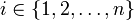$i \in \{ 1,2,\dots,n\}$, we define the partial derivative$f_{x_i}(a_1,a_2,\dots,a_n)$, also denoted$f_i(a_1,a_2,\dots,a_n)$, as follows:

• First, consider the intersection of the graph of$f$ with the plane given by the set of$n - 1$ equations$x_j = a_j$ for all$j \ne i$. This is a plane parallel to the$x_ix_{n+1}$-plane.
• In this plane, consider the slope of the tangent line at$x_i =a_i$. This is the value of the partial derivative.

Suppose$f$ is a function of multiple variables$x_1,x_2,\dots,x_n$ and suppose$(a_1,a_2,\dots,a_n)$ is a point in the domain of$f$. We say that$f$ is differentiable at$(a_1,a_2,\dots,a_n)$ if the gradient vector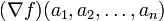$(\nabla f)(a_1,a_2,\dots,a_n)$ exists. This is equivalent to the graph of the function having a well defined tangent hyperplane at the point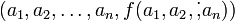$(a_1,a_2,\dots,a_n,f(a_1,a_2,\dot,a_n))$. The equation of the tangent hyperplane is given by:$x_{n+1} - f(a_1,a_2,\dots,a_n) = (\nabla f)(a_1,a_2,\dots,a_n) \cdot (\langle x_1,x_2,\dots,x_n\rangle - \langle a_1,a_2,\dots,a_n \rangle)$